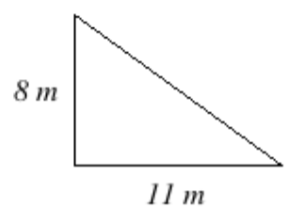# 2.4: Exercises

$$\newcommand{\vecs}{\overset { \rightharpoonup} {\mathbf{#1}} }$$ $$\newcommand{\vecd}{\overset{-\!-\!\rightharpoonup}{\vphantom{a}\smash {#1}}}$$$$\newcommand{\id}{\mathrm{id}}$$ $$\newcommand{\Span}{\mathrm{span}}$$ $$\newcommand{\kernel}{\mathrm{null}\,}$$ $$\newcommand{\range}{\mathrm{range}\,}$$ $$\newcommand{\RealPart}{\mathrm{Re}}$$ $$\newcommand{\ImaginaryPart}{\mathrm{Im}}$$ $$\newcommand{\Argument}{\mathrm{Arg}}$$ $$\newcommand{\norm}{\| #1 \|}$$ $$\newcommand{\inner}{\langle #1, #2 \rangle}$$ $$\newcommand{\Span}{\mathrm{span}}$$ $$\newcommand{\id}{\mathrm{id}}$$ $$\newcommand{\Span}{\mathrm{span}}$$ $$\newcommand{\kernel}{\mathrm{null}\,}$$ $$\newcommand{\range}{\mathrm{range}\,}$$ $$\newcommand{\RealPart}{\mathrm{Re}}$$ $$\newcommand{\ImaginaryPart}{\mathrm{Im}}$$ $$\newcommand{\Argument}{\mathrm{Arg}}$$ $$\newcommand{\norm}{\| #1 \|}$$ $$\newcommand{\inner}{\langle #1, #2 \rangle}$$ $$\newcommand{\Span}{\mathrm{span}}$$$$\newcommand{\AA}{\unicode[.8,0]{x212B}}$$

1. Classify the angle below as acute, obtuse, or right.2. Classify the angle below as acute, obtuse, or right.3. Classify the angle shown as Acute, Obtuse, or Right4. Use the picture below to answer the following questions. Note, ∠COD is a right angle.a) Which angle is supplementary to ∠BOC?

b) Which angle is complementary to ∠BOC?

c) What is the measure of ∠EOF?

d) What is the measure of ∠AOE?

e) What is the measure of ∠BOF?

5. Find the unknown angle measure.6. Find the unknown angle measure.7. Find the unknown angle measure.8. Find the unknown angle measure.9. Find the unknown angle measures.10. Find the unknown angle measure.11. Find the length of the hypotenuse of the given right triangle pictured below. Round to two decimal places.12. Find the length of the leg $$x$$. Enter the exact value, not a decimal approximation.13. Find the perimeter of the figure pictured below.14. Find the perimeter of the rectangle pictured below.15. Find the perimeter of the parallelogram shown below.16. Find the circumference of the circle pictured below. Round your answer to the nearest hundredth.17. Find the circumference of the circle pictured below. Round your answer to the nearest hundredth.18. Find the area of the rectangle pictured below.19. Find the area of the figure pictured below and state the correct units.20. Find the area of the parallelogram shown below.21. The area of a triangle can be found using the formula: $$\text{Area } = \dfrac{1}{2} \cdot \text{base} \cdot \text{height}$$. Find the area of the triangle pictured below, where the measurements are given in meters (m).22. Find the area of the circle pictured below. Round your answer to the nearest hundredth.24. Match the formula for each volume to the figure to which it applies.

Figure Volume

________ Right Circular Cylinder A. $$V = \pi r^2 h$$

________ Rectangular Solid B. $$V = \dfrac{4}{3} \pi r^3$$

________ Sphere C. $$V = l$$

25. The volume of a cylinder with height $$h$$ and radius $$r$$ can be found using the formula $$V = \pi r^2 h$$.

Sketch a cylinder with radius $$7$$ feet and height $$4$$ feet, then find the volume and select the correct units. Round your answer to the nearest tenth.

26. The volume of a cone with height $$h$$ and radius $$r$$ can be found using the formula $$V = \dfrac{1}{3} \pi r^2 h$$.

Sketch a cone with radius $$9$$ feet and height $$3$$ feet, then find the volume and select the correct units. Round your answer to the nearest tenth.

27. A sports ball has a diameter of $$26 \text{ cm}$$. Find the volume of the ball and select the correct units. Round your answer to 2 decimal places.

2.4: Exercises is shared under a CC BY-NC-SA license and was authored, remixed, and/or curated by LibreTexts.